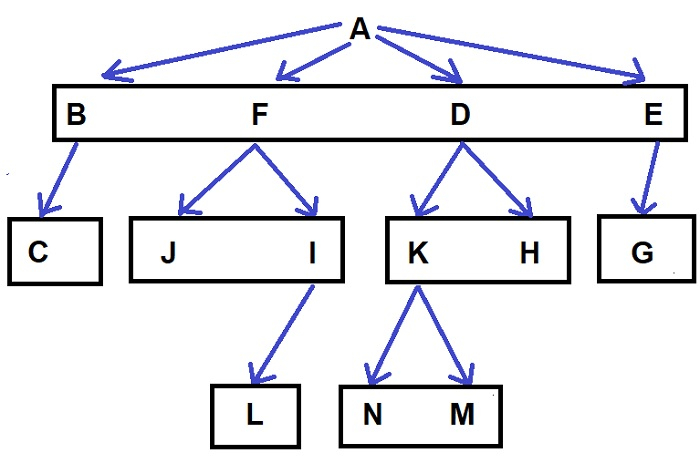# Find the Number of Ways to Traverse an N-ary Tree using C++

Given an N-ary tree and we are tasked to find the total number of ways to traverse this tree, for example −For the above tree, our output will be 192.

For this problem, we need to have some knowledge about combinatorics. Now in this problem, we simply need to check all the possible combinations for every path and that will give us our answer.

## Approach to Find the Solution

In this approach, we simply need to perform a level order traversal and check the number of children each node has and then simply multiply its factorial to the answer.

## Example

C++ Code for the Above Approach

#include<bits/stdc++.h>
using namespace std;
struct Node{ // structure of our node
char key;
vector<Node *> child;
};
Node *createNode(int key){ // function to initialize a new node
Node *temp = new Node;
temp->key = key;
return temp;
}
long long fact(int n){
if(n <= 1)
return 1;
return n * fact(n-1);
}
int main(){
Node *root = createNode('A');
(root->child).push_back(createNode('B'));
(root->child).push_back(createNode('F'));
(root->child).push_back(createNode('D'));
(root->child).push_back(createNode('E'));
(root->child->child).push_back(createNode('K'));
(root->child->child).push_back(createNode('J'));
(root->child->child).push_back(createNode('G'));
(root->child->child).push_back(createNode('C'));
(root->child->child).push_back(createNode('H'));
(root->child->child).push_back(createNode('I'));
(root->child->child->child).push_back(createNode('N'));
(root->child->child->child).push_back(createNode('M'));
(root->child->child->child).push_back(createNode('L'));
queue<Node*> q;
q.push(root);
long long ans = 1;
while(!q.empty()){
auto z = q.front();
q.pop();
ans *= fact(z -> child.size());
cout << z->child.size() << " ";
for(auto x : z -> child)
q.push(x);
}
cout << ans << "\n";
return 0;
}

## Output

4 1 2 2 1 0 0 1 2 0 0 0 0 0 192

## Explanation of the Above Code

In this approach, we apply BFS(Breadth-First Search) or level order traversal and check the number of children each node has. Then, multiply the factorial of that number to our answer.

## Conclusion

This tutorial finds several ways to traverse N-ary tree combinatorics and by applying BFS. We also learned the C++ program for this problem and the complete approach we solved.

We can write the same program in other languages such as C, java, python, and other languages. We hope you find this tutorial helpful.